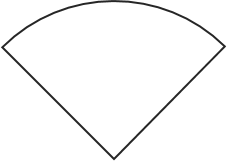# Sector Area Calculator

This sector area calculator can help – as you can guess – in determining the area of a sector. Simply enter the radius and the angle and the sector area appears in no time. If you’re interested in knowing how to find the area of a sector, keep reading and you will find a detailed description of the sector area formula.

## What is a ‘sector’?

A sector of a circle is essentially a proportion of the circle that is enclosed by two radii and an arc.## Sector area formula

The equation for calculating the area of a sector is as follows:

`area = πr2 * (A / 360)`

where r is the radius of the circle and A is the angle of the arc in degrees.

## How to find the area of a sector?

You can use the sector area calculator to find the area of a sector: